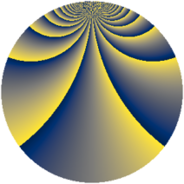# Properties

 Label 2001.2.buLevel $2001$ Weight $2$ Character orbit 2001.bu Rep. character $\chi_{2001}(10,\cdot)$ Character field $\Q(\zeta_{308})$ Dimension $14400$ Sturm bound $480$

# Related objects

## Defining parameters

 Level: $$N$$ $$=$$ $$2001 = 3 \cdot 23 \cdot 29$$ Weight: $$k$$ $$=$$ $$2$$ Character orbit: $$[\chi]$$ $$=$$ 2001.bu (of order $$308$$ and degree $$120$$) Character conductor: $$\operatorname{cond}(\chi)$$ $$=$$ $$667$$ Character field: $$\Q(\zeta_{308})$$ Sturm bound: $$480$$

## Dimensions

The following table gives the dimensions of various subspaces of $$M_{2}(2001, [\chi])$$.

Total New Old
Modular forms 29280 14400 14880
Cusp forms 28320 14400 13920
Eisenstein series 960 0 960

## Trace form

 $$14400q + 8q^{2} - 8q^{8} + O(q^{10})$$ $$14400q + 8q^{2} - 8q^{8} - 224q^{16} - 8q^{18} - 44q^{19} - 4q^{23} - 120q^{25} - 112q^{26} - 100q^{29} - 48q^{31} + 168q^{32} - 112q^{35} - 240q^{36} + 72q^{39} + 8q^{41} - 88q^{43} - 308q^{44} + 128q^{46} + 88q^{47} - 48q^{48} - 336q^{49} - 292q^{50} - 616q^{51} + 224q^{52} + 76q^{55} - 220q^{56} + 68q^{58} + 16q^{59} - 56q^{69} + 152q^{70} - 112q^{71} - 64q^{72} - 4q^{73} - 220q^{74} - 16q^{75} - 120q^{77} - 240q^{81} - 48q^{82} + 84q^{85} - 8q^{87} + 704q^{88} - 264q^{89} + 32q^{94} - 336q^{95} - 264q^{97} + 672q^{98} + O(q^{100})$$

## Decomposition of $$S_{2}^{\mathrm{new}}(2001, [\chi])$$ into newform subspaces

The newforms in this space have not yet been added to the LMFDB.

## Decomposition of $$S_{2}^{\mathrm{old}}(2001, [\chi])$$ into lower level spaces

$$S_{2}^{\mathrm{old}}(2001, [\chi]) \cong$$ $$S_{2}^{\mathrm{new}}(667, [\chi])$$$$^{\oplus 2}$$